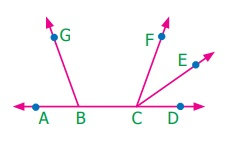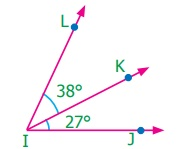Home | | Maths 7th Std | Exercise 5.1

# Exercise 5.1

7th Maths : Term 1 Unit 5 : Geometry : adjacent angles, linear pair of angles and vertically opposite angles : Exercise 5.1 : Text Book Back Exercises Questions with Answers, Solution

Exercise 5.1

1. Name the pairs of adjacent angles.The pairs of adjacent angle are

ABG and GBC, BCF and FCE

FCE and ECD, ACF and FCE

ACF and ECD

2. Find the angle JIL from the given figure.JIL = 38° + 27° = 65°

3. Find the angle GEH from the given figure.GEH = 120° – 34° = 86°

4. Given that AB is a straight line. Calculate the value of x° in the following cases.i) AOC + BOC = 180°

72° + x° = 180°

x° = 180° – 72° = 108°

ii) AOC + BOC = 180°

3x° + 42° = 180°

3x° = 180° – 42o = 138o

x° = 138° / 3 = 46o

x = 46°

iii) AOC + BOC = 180°

4x° + 2x° = 180°

6x° = 180°

x° = 180° / 6o = 30o

x° = 30°

5. One angle of a linear pair is a right angle. What can you say about the other angle?

The other angle is also a right angle.

6. If the three angles at a point are in the ratio 1 : 4 : 7, find the value of each angle?

Three angles are in the ratio 1:4:7

Let the three angles be x°, 4x°, and 7x°

Sum of the three angles at a point x° + 4x° + 7x° = 360°

12x° = 360°

x° = 360° / 12 = 30o

x° = 30o

4x° = 4 × 30° = 120°

7x° = 7 × 30° = 120°

The three angles are 30°, 120°, 210°

7. There are six angles at a point. One of them is 45° and the other five angles are all equal. What is the measure of all the five angles?

The sum of six angles at a point = 360°

One angle = 45°

The other five angles = 315°

The measure of each angles = 315° / 5 = 63°

8. In the given figure, identify(i) any two pairs of adjacent angles.

(ii) two pairs of vertically opposite angles.

(i) any two pairs of adjacent angles.

PQT and TQS

TQS and SQR

SQR and RQU

RQU and PQU

(ii) two pairs of vertically opposite angles.

Vertically opposite angles

PQU and TQR

PQT and UQR

9. The angles at a point are x°, 2x°, 3x°, 4x° and 5x°. Find the value of the largest angle?

Sum of the angles at a point

x° + 2x° + 3x° + 4x° + 5x° = 360°

15x° = 360°

x° = 360° / 15 = 24°

x = 24°

The largest angle = 5x° = 5 × 24° = 120o

10. From the given figure, find the missing angle.ROQ and POS are vertically opposite angles. They are equal.

ROQ =105°

POS = x° = 105°

11. Find the angles x° and y° in the figure shown.3x° + x° = 180°

4x° = 180°

x° = 180/4 = 45o

xo = 45°

x° = 45°

3x° = 3 × 45° = 135o

y° = 3x° (vertically opposite angles)

y°= 135°

12. Using the figure, answer the following questions.

(i) What is the measure of angle x°?

(ii) What is the measure of angle y°?The measure of x = 125° (vertically opposite angles)

x° + y° = 180°

125o + yo = 180°

yo = 180° – 125° = 55°

x° = 125°

y° = 55°

Objective type questions

(i) no common interior, no common arm, no common vertex

(ii) one common vertex, one common arm, common interior

(iii) one common arm, one common vertex, no common interior

(iv) one common arm, no common vertex, no common interior

Answer : iii) one common arm, one common vertex, no common interior

14. In the given figure the angles 1 and 2 are(i) opposite angles

(iii) Linear pair

(iv) Supplementary angles

15. Vertically opposite angles are

(i) not equal in measure

(ii) complementary

(iii) supplementary

(iv) equal in measure

Answer : iv) Equal in measure

16. The sum of all angles at a point is

(i) 360°

(ii) 180°

(iii) 90°

(iv) 0°

17. The measure of BOC is(i) 90°

(ii) 180°

(iii) 80°

(iv) 100°

Exercise 5.1

1. The pairs of adjacent angle are ABG and GBC , BCF and FCE , FCE and ECD , ACF and FCE , ACF and ECD

2. 65°

3. 86°

4. (i) 108° (ii) 46° (iii) 30°

5. The other angle is also a right angle.

6. 30° , 120°, 210°

7. 63°

8. Adjacent angles: PQU and PQT , and TQS , TQS and SQR , SQR and RQU , RQU and PQU (all possible answers)

Opposite pairs: PQU and TQR , PQT and UQR

9. 120°

10. 105°

11. 45°, 135°

12. (i) 125° (ii) 55°

Objective type questions

13. (iii) one common arm, one common vertex, no common interior

14. (iii) linear pair

15. (iv) equal in measure

16. (i) 360°

17. (ii) 80°

Tags : Questions with Answers, Solution | Geometry | Term 1 Chapter 5 | 7th Maths , 7th Maths : Term 1 Unit 5 : Geometry
Study Material, Lecturing Notes, Assignment, Reference, Wiki description explanation, brief detail
7th Maths : Term 1 Unit 5 : Geometry : Exercise 5.1 | Questions with Answers, Solution | Geometry | Term 1 Chapter 5 | 7th Maths